# Chebyshev polynomials

of the first kind

Polynomials that are orthogonal on the intervalwith the weight function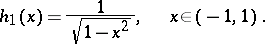For the standardized Chebyshev polynomials one has the formula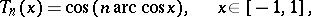and the recurrence relationby which one can determine the sequence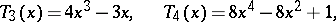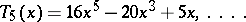The orthonormalized Chebyshev polynomials are: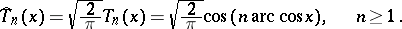The leading coefficient of, for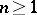, is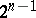. Hence Chebyshev polynomials with leading coefficient 1 are defined by the formula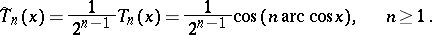The zeros of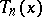, given byfrequently occur as interpolation nodes in quadrature formulas. The polynomialis a solution of the differential equation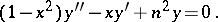The polynomialsdeviate as least as possible from zero on the interval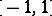, that is, for any other polynomial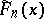of degreewith leading coefficient 1 one has the following condition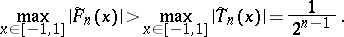On the other hand, for any polynomialof degreeor less and satisfying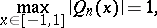one has, for any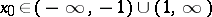, the inequality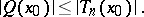If a functionis continuous on the intervaland if its modulus of continuity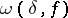satisfies the Dini condition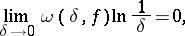then this function can be expanded in a Fourier–Chebyshev series,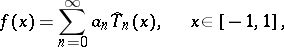which converges uniformly on. The coefficients in this series are defined by the formulaIf the functionis-times continuously differentiable onand if its-th derivative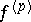satisfies a Lipschitz condition of order, i.e.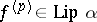, then one has the inequalitywhere the constant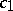does not depend onand.

Chebyshev polynomials of the second kind are defined by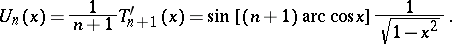These polynomials are orthogonal on the intervalwith weight function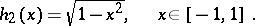For any polynomial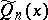with leading coefficient 1 one has the inequality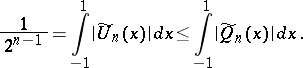The Chebyshev polynomials were introduced in 1854 by P.L. Chebyshev (cf. ). Both systems of Chebyshev polynomials are special cases of ultraspherical polynomials and Jacobi polynomials.